GFG App
Open AppBrowser
Continue

# Class 9 RD Sharma Solutions – Chapter 14 Quadrilaterals- Exercise 14.4 | Set 2

### (ii) ABCP is a parallelogram.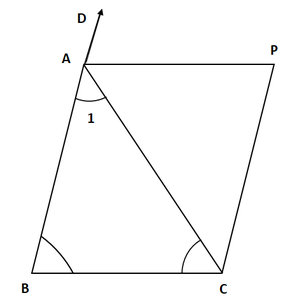Solution:

From the question it is given that

AB = AC, CD ∥ BA and AP is the bisector of exterior ∠CAD of ΔABC

(i) So, we have

AB = AC    [Given]

∠ACB = ∠ABC      [Because opposite angles of equal sides of triangle are equal]

Now, ∠CAD = ∠ABC + ∠ACB

= 2∠PAC = 2∠ACB

=∠PAC = ∠ACB

Hence proved

(ii) Now, we have

∠PAC = ∠BCA  [Proved above]

AP ∥ BC and CP ∥ BA     [Given]

Hence, ABCP is a parallelogram.

### Question 12. ABCD is a kite having AB = AD and BC = CD. Prove that the figure found by joining the mid points of the sides, in order, is a rectangle.

Solution: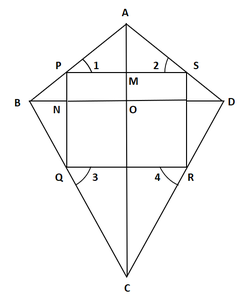From the question it is given that

A kite ABCD having AB = AD and BC = CD.

Join PQ, QR, RS and SP

So, P, Q, R, S are the mid-points of sides AB, BC, CD and DA.

Now e have to prove that PQRS is a rectangle.

Proof:

In ∆ABC,

It is given that P and Q are the mid-points of AB and BC

So from mid point theorem

PQ ∥ AC and PQ = (1/2) AC        …. (i)

It is given that R and S are the mid-points of CD and AD

So from mid point theorem

RS ∥ AC and RS = (1/2) AC     …. (ii)

From eq(i) and (ii) we have

PQ ∥ RS and PQ = RS

So, PQRS is a parallelogram.

Now, we prove that in parallelogram PQRS on angle is a right angle.

=  AP = AS      … (iii)

=  ∠1 = ∠2             …. (iv)

Now, in ΔPBQ and ΔSDR, we have

PB = SD              [AD = AB ⇒ (1/2) AD = (1/2) AB]

BQ = DR            [Since PB = SD]

And PQ = SR     [Since, PQRS is a parallelogram]

So, by SSS criterion of congruence, we have

ΔPBQ ≅ ΔSDR

By c.p.c.t

= ∠3 = ∠4

Now, ∠3 + ∠SPQ + ∠2 = 180°

And ∠1 + ∠PSR + ∠4 = 180°

∠3 + ∠SPQ + ∠2 = ∠1 + ∠PSR + ∠4

= ∠SPQ = ∠PSR               [∠1 = ∠2 and ∠3 = ∠4]

From the figure we know that transversal PS cuts parallel lines SR and PQ at S and P

So, ∠SPQ + ∠PSR = 180°

= 2∠SPQ = 180°

= ∠SPQ = 90°              [∠PSR = ∠SPQ]

Hence, PQRS is a parallelogram and∠SPQ = 90°.

### Question 13. Let ABC be an isosceles triangle in which AB = AC. If D, E, F be the mid points of the, sides BC, CA and AB respectively, show that the segment AD and EF bisect each other at right angles.

Solution: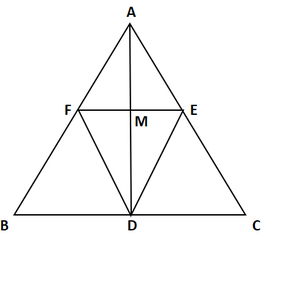To prove : segment AD and EF bisect each other at right angles.

Proof:

In ΔABC,

It is given that D, E and F are mid-points of sides BC, CA and AB

So from mid point theorem

AB ∥ DE and AC ∥ DF

AF ∥ DE and AE ∥ DF

ABDE is a parallelogram.

AF = DE and AE = DF           [Because opposite sides of parallelogram are congruent]

So from mid point theorem

(1/2) AB = DE and (1/2) AC = DF

DE = DF

AE = AF = DE = DF

ABDF is a rhombus.

AD and FE bisect each other at right angle.

Hence Proved

### Question 14. ABC is a triangle. D is a point on AB such that AD = (1/4) AB and E is a point on AC such that AE = (1/4) AC. Prove that DE = (1/4) BC.

Solution: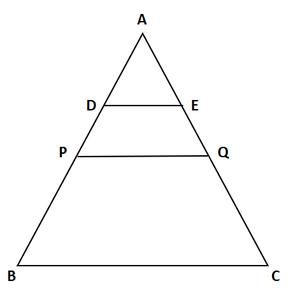To prove : DE = (1/4) BC.

Proof:

In ΔABC,

D is a point on AB so,

AB and E is a point on AC so,

AE = (1/4) AC.

Let us assume P and Q be the mid-points of AB and AC

Then PQ ∥ BC

So from mid point theorem

PQ = (1/2) BC   …. (i)

In ΔAPQ,

D and E are the mid-points of AP and AQ

So from mid point theorem

(1/2) DE ∥ PQ, and DE = (1/2) PQ   …. (ii)

From eq(i) and (ii) we know that,

DE = (1/2) PQ = (1/2) ((1/2) BC)

DE = (1/2) BC

Hence proved.

### Question 15. In Figure, ABCD is a parallelogram in which P is the mid-point of DC and Q is a point on AC such that CQ = (1/2) AC. If PQ produced meets BC at R, prove that R is a mid-point of BC.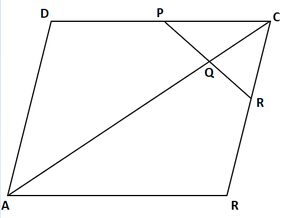Solution:

Now join B and D.

Suppose AC and BD intersect at O.

So from mid point theorem

Then OC = (1/2) AC

Now,

CQ = (1/4) AC

⇒ CQ = 1/2((1/2) AC)

= (1/2) OC

In ΔDCO,

PQ ∥ DO           [Because P and Q are mid points of DC and OC]

Similarly in ΔCOB,

QR ∥ OB                       [Because Q is the mid-point of OC]

R is the mid-point of BC.

Hence Proved

### (ii) PR = (1/2) AC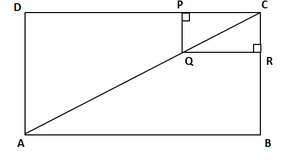Solution:

It is given that Q is the mid-point of AC such that PQ ∥ AD

So, P is the mid-point of DC.

From mid point theorem

DP = DC

Hence Proved

(ii) Similarly,

PR = (1/2) BD         [R is the mid-point of BC]

PR = (1/2) AC        [Diagonal of rectangle are equal, BD = AC]

Hence Proved

### Question 17. ABCD is a parallelogram; E and f are the mid-points of AB and CD respectively. GH is any line intersecting AD, EF and BC at G, P and H respectively. Prove that GP = PH.

Solution: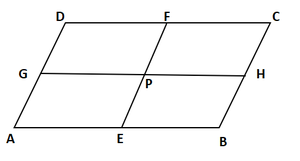From the question it is given that

ABCD is a parallelogram

E and F are mid-points of AB and CD

AE = BE = (1/2) AB

And CF = DF = (1/2) CD

But, AB = CD

(1/2)AB = (1/2) CD

BE = CF

Also, BE ∥ CF [∴ AB ∥ CD]

Hence, BEFC is a parallelogram

BC ∥ EF and BE = PH  …. (i)

Now, BC ∥ EF

Hence, AEFD is a parallelogram.

AE = GP

So, AE = BF                              [E is the mid-point of AB]

GP = PH.

Hence Proved.

### Question 18. BM and CN are perpendiculars to a line passing through the vertex A of triangle ABC. If L is the mid-point of BC, prove that LM = LN.

Solution: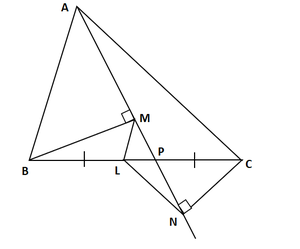To prove: LM = LN

Draw LS perpendicular to line MN.

The lines BM, LS and CN are perpendicular on MN line and parallel to each other.

According to Intercept Theorem,

From above figure we know that, MB, LS and NC are three parallel

lines and the two transversal lines are MN and BC.

BL = LC                                        [L is mid-point of BC]

We know that,

MS = SN  ….. (i)                                    [By Intercept Theorem]

Now, In ΔMLS and ΔLSN

MS = SN                               [From equation (i)]

∠LSM = ∠LSN = 90°           LS ⊥ MN]

And SL = LS                        [common side]

By SAS Congruency Rule

ΔMLS ≅ ΔLSN

Hence, by c.p.c.t

LM = LN

Hence Proved

### Question 19. Show that, the line segments joining the mid-points of opposite sides of a quadrilateral bisects each other.

Solution: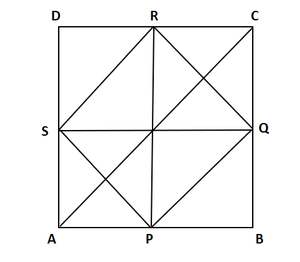Let us considered ABCD is a quadrilateral in which

P, Q, R and S are mid-points of sides AB, BC, CD and DA

Now, join PQ, QR, RS, SP, and BD

In ΔABD,

It is given that P and S are mid-points of sides AB and DA

So from mid point theorem

SP ∥ BD and SP = (1/2) BD          …. (i)

In ΔBCD

It is given that Q and R are mid-points of sides BC and CD

So from mid point theorem

QR ∥ BD and QR = (1/2) BD          …. (ii)

Now,

From eq(i) and (ii)

SP ∥ QR and SP = QR

So, SPQR is a parallelogram                    [ Diagonals of a parallelogram bisect each other]

Hence Proved, PR and QS bisect each other.

### Question 20. Fill in the blanks to make the following statements correct:

(i) The triangle formed by joining the mid-points of the sides of an isosceles triangle is ____________.

(ii) The triangle formed by joining the mid-points of the sides of a right triangle is ____________.

(iii) The figure formed by joining the mid-points of consecutive sides of a quadrilateral is ____________.

Solution:

(i) Isosceles

(ii) Right triangle

(iii) Parallelogram

My Personal Notes arrow_drop_up